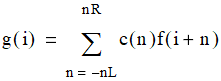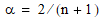# NMath User's Guide

14.1 Moving Window Filtering (.NET, C#, CSharp, VB, Visual Basic, F#)

Class replaces data points f(i) with a linear combination, g(i), of the data points immediately to the left and right of f(i), based on a given set of coefficients, c, to use in the linear combination. The neighboring points are determined by the number of points to the left, nL, and the number of points to the right, nR:MovingWindowFilter extends class CorrelationFilter which provides basic correlation services.

Creating Moving Window Filter Objects

A MovingWindowFilter instance is constructed from the number of points to the left and right of the input point, and the coefficients of the linear combination.

For example, this code constructs an asymmetric moving window filter of length 5:

Code Example – C# signal filtering

```int numberLeft = 1;
```
```int numberRight = 3;
```
```var filterCoefficients = new DoubleVector(5, 0.20);
```
```var filter = new MovingWindowFilter( numberLeft, numberRight,
```
```  filterCoefficients );
```

Code Example – VB signal filtering

```Dim NumberLeft = 1
```
```Dim NumberRight = 3
```
```Dim FilterCoefficients As New DoubleVector(5, 0.2)
```
```Dim Filter As New MovingWindowFilter(NumberLeft, NumberRight,
```
```  FilterCoefficients)
```

An InvalidArgumentException is raised if the length of the coefficient vector is not equal to numberLeft + numberRight + 1.

Static class methods are provided for generating coefficient vectors of three common types:

MovingAverageCoefficients() constructs a coefficient vector that implements a moving average filter.

ExponentiallyWeightedMovingAverageCoefficients() constructs a coefficient vector of exponentially weighted moving average (EWMA) coefficients of the specified length. As the number of EWMA coefficients increases, the filter captures at most %86.47 of the total weight due to the finite length of the filter. The filter length n and the exponential weightare related by.

SavitzkyGolayCoefficients() constructs a coefficient vector that implements a Savitzky-Golay smoothing filter (also known as least-squares, or DIgital Smoothing POlynomial, DISPO). The filter coefficients are chosen such that the filtered point is the value of an approximating polynomial of the specified order, typically quadratic or quartic. The polynomial is fit using a least squares algorithm.

For example, the following code constructs a moving average filter to replace each input data point with the average of it's value and the surrounding points:

Code Example – C# signal filtering

```int numberLeft = 4;
```
```int numberRight = 5;
```
```DoubleVector filterCoefficients =
```
```  MovingWindowFilter.MovingAverageCoefficients( numberLeft,
```
```    numberRight );
```
```var filter = new MovingWindowFilter( numberLeft, numberRight,
```
```  filterCoefficients );
```

Code Example – VB signal filtering

```Dim NumberLeft = 4
```
```Dim NumberRight = 5
```
```Dim FilterCoefficients As DoubleVector =
```
```  MovingWindowFilter.MovingAverageCoefficients(NumberLeft,
```
```    NumberRight)
```
```Dim Filter As New MovingWindowFilter(NumberLeft, NumberRight,
```
```  FilterCoefficients)
```

This code creates a Savitzky-Golay filter that replaces each input data point with the value of a fourth degree polynomial fit through the input value and it's surrounding points:

Code Example – C# signal filtering

```int numberLeft = 3;
```
```int numberRight = 3;
```
```int degree = 4;
```
```DoubleVector filterCoefficients =
```
```  MovingWindowFilter.SavitzkyGolayCoefficients( numberLeft,
```
```    numberRight, degree );
```
```var filter = new MovingWindowFilter( numberLeft, numberRight,
```
``` filterCoefficients );
```

Code Example – VB signal filtering

```Dim NumberLeft = 3
```
```Dim NumberRight = 3
```
```Dim Degree = 4
```
```Dim FilterCoefficients As DoubleVector =
```
```  MovingWindowFilter.SavitzkyGolayCoefficients(NumberLeft,
```
```    NumberRight, Degree)
```
```Dim Filter As New MovingWindowFilter(NumberLeft, NumberRight,
```
```  FilterCoefficients)
```

This code creates an exponential moving average filter of length 18:

Code Example – C# signal filtering

```int n = 18;
```
```DoubleVector filterCoefficients =
```
``` MovingWindowFilter.ExponentiallyWeightedMovingAverageCoefficients(
n );

```

```var EWMAfilter = new MovingWindowFilter( 0,
```
```  coef.filterCoefficients - 1, filterCoefficients );
```

Code Example – VB signal filtering

```Dim N = 18
```
```Dim FilterCoefficients As DoubleVector =
```
```
```
``` MovingWindowFilter.ExponentiallyWeightedMovingAverageCoefficients(
```
```  N)

```

```Dim EWMAfilter As New MovingWindowFilter(0,
```
```  Coef.FilterCoefficients - 1, filterCoefficients)
```

After construction, the SetFilterParameters() method can be used to reset the filter parameters on a filter instance:

Code Example – C# signal filtering

```filter.SetFilterParameters( numberLeft, numberRight,
```
```  filterCoefficients );
```

Code Example – VB signal filtering

```Filter.SetFilterParameters(NumberLeft, NumberRight,
```
```  FilterCoefficients)
```

Moving Window Filter Properties

Once constructed, a MovingWindowFilter object provides the following read-only properties:

NumberLeft gets the number of points to the left for the filter window.

NumberRight gets the number of points to the right for the filter window.

WindowWidth gets the width of the moving window (equal to NumberLeft + NumberRight + 1).

NumberOfCoefficients gets the number of filter coefficients (equal to WindowWidth).

Coefficients gets the vector of filter coefficients.

Filtering Data

The Filter() method on MovingWindowFilter applies a filter to a given data set using the specified boundary option.

The MovingWindowFilter.BoundaryOption enumeration specifies options for handling the boundaries in a moving window filter, where the filter does not complete overlap with the data:

BoundaryOption.PadWithZeros adds NumberLeft zeros to the beginning of the data to be filtered and NumberRight zeros to end.

BoundaryOption.DoNotFilterBoundaryPoints specifies that the first NumberLeft and the last NumberRight data will not be filtered.

For example, the following code constructs a noisy cosine signal, and then filters the data:

Code Example – C# signal filtering

```var rng = new RandGenNormal();
```
```var noisySignal = new DoubleVector( length );
```
```for ( int i = 0; i < length; i++ )
```
```{
```
```  noisySignal[i] = Math.Cos( .2*i ) + rng.Next();
```
```}

```

```DoubleVector filteredSignal = filter.Filter( noisySignal,
```
```  MovingWindowFilter.BoundaryOption.PadWithZeros );
```

Code Example – VB signal filtering

```Dim RNG As New RandGenNormal()
```
```Dim NoisySignal As New DoubleVector(Length)
```
```For I As Integer = 0 To Length - 1
```
```  NoisySignal(I) = Math.Cos(0.2 * I) + RNG.Next()
```
```Next

```

```Dim FilteredSignal As DoubleVector = Filter.Filter(NoisySignal,
```
```  MovingWindowFilter.BoundaryOption.PadWithZeros)
```

Top

Top